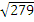## Monday, 27 April 2015

### SBI PO Quantitative Aptitude Quiz -11

Direction (1 - 5): What will come in place of the question mark (?) in the following questions?

1) 17 2) 19 3)4) 289 5) None of these

2. 93 x 812 ÷ 273 = (3)?

1) 3 2) 4 3) 5 4) 6 5) None of these

3. 572 ÷ 26 x 12 – 200 = (2)?

1) 5 2) 6 3) 7 4) 8 5) None of these

5. 36% of 245 – 40% of 210 = 10 -?

1) 4.2 2) 6.8 3) 4.9 4) 5.6 5) None of these

6. Rita invested 25% more than Sunil. Sunil invested 30% less than Abhinav who invested Rs. 6,000. What is the respective ratio between the amount that Rita invested and the total amount invested by all of them together?

(1) 35 : 104 (2) 13 : 29 (3) 101 : 36 (4) 35 : 103 (5) None of these

7. A man gets a simple interest of Rs. 1,000 on a certain principal at the rate of 5 p.c.p.a. in 4 years. What compound interest will the man get on twice the principal in two years at the same rate?

(1) Rs. 1,050 (2) Rs. 1,005 (3) Rs. 11,025 (4) Rs. 10,125 (5) None of these

8. The perimeter of a square is equal to twice the perimeter of a rectangle of length 8 cms. And breadth 7 cms. What is the circumference of a semicircle whose diameter is equal to the side of the square ? (Rounded off to the' two decimal places)

(1) 38.57 cms. (2) 23.57 cms. (3) 42.46 cms. (4) 47.47 cms. (5) None of these

9. Radha's present age is three years less than twice her age 12 years ago. Also the respective ratio between Raj's present age and Radha's present age is 4 : 9. What will be Raj's age after 5 years?

(1) 12 years (2) 7 years (3) 21 years (4) Cannot be determined (5) None of these

10. The respective ratio between the speeds of a car, a train and a bus is 5 : 9 : 4. The average speed of the car, the bus and the train is 72 kmph together. What is the average speed of the car and the train together?

(1) 82 kmph (2) 78 kmph (3) 84 kmph (4) Cannot be determined (5) None of these

2. 3; (32)3 x (34)2 ÷ (33)3 = 3?

= 36 x 38 ÷ 39 = 3?

= 36+8-9 = 3?

= 3? = 35 = ? = 5

= 264 – 200 = 2?

= 64 = 2?

= 26 = 2? = ? = 6

= 10 - ?

= 88.20 – 84 = 10 - ?

= 4.20 = 10 - ?

= ? = 10 – 4.20 = 5.8

6. 4; Abhinav’s investment = Rs. 6000

= Rs. 4200

= Rs. 5250

∴ Required ratio

= 5250 : (6000 + 4200 + 5250)

= 5250 : 15450 = 35 : 103

Case II

Principal = Rs. 10000

8. 1; Perimeter of square

= 2 x Perimeter of rectangle

= 2 x 2 (8+7) = 60 cm.

∴ Diameter of semi-circle = 15 cm.

∴ Circumferences of semi-circle

9. 5; Let Radha’s present age = x years.

∴ x = 2 (x - 12) – 3

= x = 2x – 24 – 3 = x = 27

= 12 years

∴ Raj’s age after 5 years

= 12 + 5 = 17 years

10. 3; 5x + 9x + 4x = 72 x 3

= 18x = 72 x 3

∴ Average speed of car and train

= 7 x 12 = 84 kmph